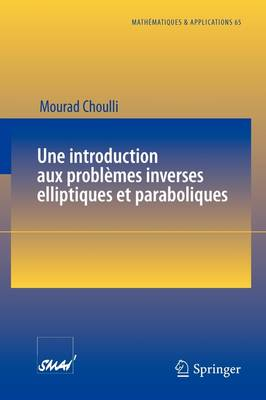•Une Introduction Aux Probl mes Inverses Elliptiques Et Paraboliques - Matha©Matiques Et Applications 65 (Paperback)

(author)
£54.99
Paperback 252 Pages / Published: 22/09/2009
• We can order this

Usually dispatched within 3 weeks

Cet ouvrage est consacr une introduction aux probl mes inverses elliptiques et paraboliques. L' objectif est de pr senter quelques m thodes r centes pour tablir des r sultats d'unicit et de stabilit . Seront trait s quelques probl mes inverses elliptiques devenus maintenant classiques, tels que la conductivit inverse, la d tection de corrosion ou de fissures et les probl mes spectraux inverses. Parmi les probl mes inverses paraboliques consid r s figurent le probl me classique de retrouver une distribution initiale de la chaleur et la localisation de sources, de chaleur ou de pollution par exemple. Les probl mes d'identification de non lin arit s seront aussi tudi s.

Cet ouvrage s'adresse tous ceux qui souhaitent s' int resser l'analyse math matique des probl mes inverses.

This book is devoted to an introduction of elliptic and parabolic inverse problems. Our goal is to present some recent methods for establishing uniqueness and stability results. We study some classical elliptic inverse problems: inverse conductivity problem, detection of corrosion or cracks and inverse spectral problems. Among the parabolic inverse problems we consider the classic problem of finding an initial distribution of heat and the location of sources. We hope that this book will interest all those who want to learn the mathematical analysis of inverse problems.

Publisher: Springer-Verlag Berlin and Heidelberg GmbH & Co. KG
ISBN: 9783642024597
Number of pages: 252
Weight: 390 g
Dimensions: 234 x 156 x 15 mm
Edition: 2009 ed.

MEDIA REVIEWS

From the reviews:

"This is a nicely written monograph which presents a number of basic results about uniqueness and stability for elliptic and parabolic inverse problems in a thorough and uniform manner, and should be of wide interest to researchers in inverse problems for partial differential equations. ... The text concludes with a collection of advanced exercises and a brief discussion of some open problems." (Paul E. Sacks, Mathematical Reviews, Issue 2010 m)

"The book is devoted to the determination of unknown coefficients or unknown - i.e. unaccessible - subdomains or parts of the boundary related to both elliptic and parabolic equations. ... In conclusion, the reviewer not only recommends this well-written book as a good trainer for young mathematicians wishing to be acquainted with inverse problems for PDEs, but also recommends it to researchers, since they can find in it a lot of stimulating ideas and indispensable techniques needed to solve nowadays inverse problems." (Alfredo Lorenzi, Zentralblatt MATH, Vol. 1192, 2010)